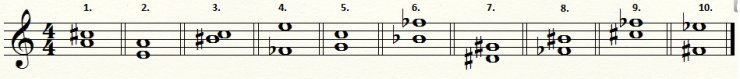# Let's Learn Music Theory 03: Intervals and Transposing

by FiniteZer0 (Apr 02, 2012)

## Introduction

In this lesson we develop and use the understanding we gained from lesson 02. Lesson 02 centered around scales, which is an essential cog in the machine of music theory.

We will learn very common music terminology and what they mean. We will also learn how to transpose melodies from one key to another.

Tones and tone combinations are a dominant theme in Music Theory, so we will gain an understanding of these tones and their relationship to one another. Of the many different relationships, the interval is the most basic.

Interval:The relationship between two tones.

We name an interval based on its diatonic number. For example F and A are two whole steps apart, so we call this interval a third.

Below I will demonstrate all of the intervals to their corresponding scale degree.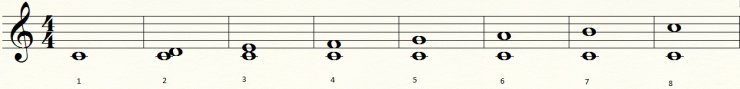An octave refers to interval 8

Interval 1 is known as unison

## Perfect, Major, and Minor Intervals

When an interval includes the tonic and a fourth or a fifth, it is known as a perfect fourth or fifth. Additionally, unison and octaves are also named perfect.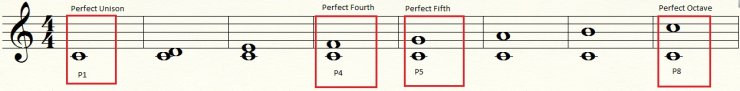When an interval contains the tonic and a second, third, sixth, or seventh of a major scale, it is named Major.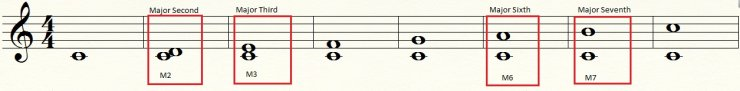When an interval becomes a half-step smaller it is called minor.

Here is a list for the standard abbreviations of the intervals and I will provide an illustration below it.

```
Name                         Abbreviation
Perfect Unison (Prime)            P1
minor second                      m2
Major second                      M2
minor third                       m3
Major third                       M3
Perfect fourth                    P4
Perfect fifth                     P5
minor sixth                       m6
Major sixth                       M6
minor seventh                     m7
Major seventh                     M7
Perfect Octave                    P8
```Consonant Intervals: Consonant intervals, at least contrapuntally, are as follows: m3, M3, P5, m6, M6, P8, and P1

Dissonant Intervals: The dissonant intervals are the following: m2, M2, P4, m7, and M7. Pretty much everything else.

Augmented Intervals: If a composer increases the distance of a Major or Perfect interval by a half-step, she creates an Augmented interval. These intervals are abbreviated with an A, for example, A5 is an Augmented fifth.

Diminished Intervals: If a composer decreases the distance of a minor or Perfect interval by a half-step, she creates a diminished interval, which is abbreviated with a d, for example, d4 is a diminished fourth.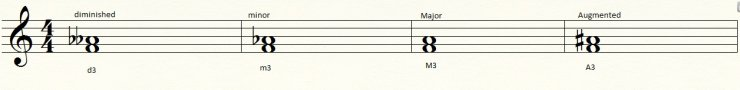Please note that an interval can be diminished or Augmented as long as it does not change the interval number.

## Enharmonic Intervals

An enharmonic interval is an interval that sounds the same but is spelled differntly, for example, F♯ and G♭ are considered enharmonic.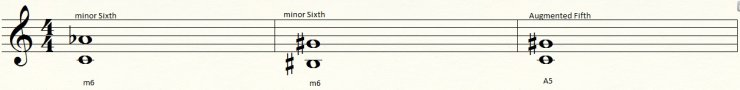If you play these notes, they should all sound the same! =D

Spelling an interval correctly is an absolute must. If a specific interval is requested, the enharmonic spelling is incorrect. If someone asks for a third above D, do not write G♭; the correct answer would be F♯. Yes G♭ and F♯ sound the same, but they have completely different spellings.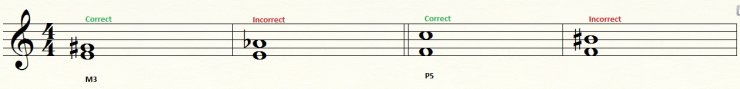The Triton: The most common enharmonic interval within an octave is the tritone. It got its name for it devides an octave in half half. Tritones are also three whole steps apart.

The tritones are written as A4 and d5.

## Inversions of Intervals

Inversion: when the lower interval becomes the higher interval and vice versa.

Here is a table that you should memorize:

```
Interval                When Inverted
Perfect                  Perfect
Major                    minor
minor                    Major
diminished               Augmented
Augmented                diminished
Unison                   Octave
Second                   Seventh
Third                    Sixth
Fourth                   Fifth
Fifth                    Fourth
Sixth                    Third
Seventh                  Second
Octave                   Unison
```

## Compound Intervals

Compound Interval: An interval is compounded if the interval exceeds an octave. If an interval is within an octave it is named simple.

Compound Intervals and their simple equivalents
```
Compound Interval          Simple Interval
M9                               M2
M10                              M3
P11                              P4
P12                              P5
M13                              M6
M14                              M7
M15                              P8
```

## The Importance of Knowing Intervals

It is necessary to develop the ability to recognize intervals as quick as possible; for if you wish to learn music theory, you will need to read and write this as quick as possible.

I will give you a list of cold hard facts that can make memorizing intervals as quick as possible.

1. 3rds, 5ths, and 7ths have intervals on both lines or spaces on a sheet of music.2. 2nds, 4ths, 6ths, and 8ths contain one line and one space or one space and one line on a sheet of music.3. 4ths, 5ths, and 8ths are perfect if the accidentals are the same.
[dot]B and F are the only exception to this rule for they are a tritone apart.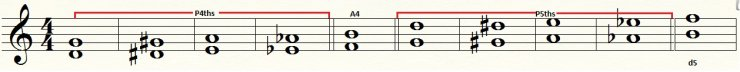4. An interval is a M2 or m7 if the accidentals are the same except for E-F and B-C.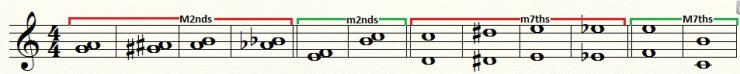5. Thirds build on C, F, and G, are Major if the accidentals are the same. Thirds built on everything else are minor if the accidentals are the same.

(Figure 3.12)

6. Sixths whose uppertones are C, F, and G are minor if their accidentals are the same. The remaining notes are Major if their accidentals are the same.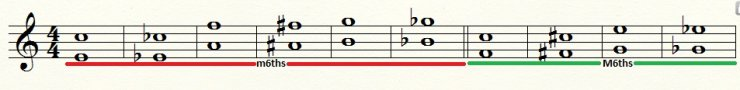7. It is possible to quickly determine other interval qualities by reducint the interval to the "same accidental" form and then noting the effect on interval size when the accidental(s) are replaced.## Harmonic and Melodic Intervals

Harmonic Intervals: Intervals are harmonic if they are played at the same time.

Melodic: Intervals are melodic if they are played immediately after each other.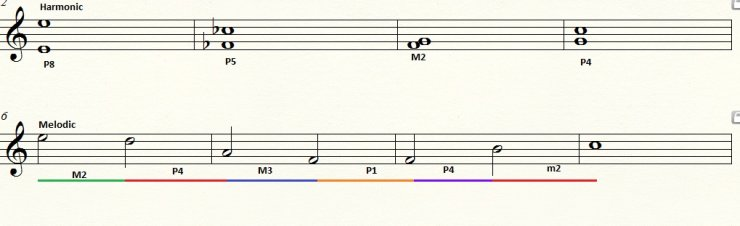)

## Transposing

There are multiple methods of transposing music. Unfortunately, in this lesson we will examine the most common method which is Interval Transposition.

Interval Transposition: Choose an interval and move all pitches accordingly.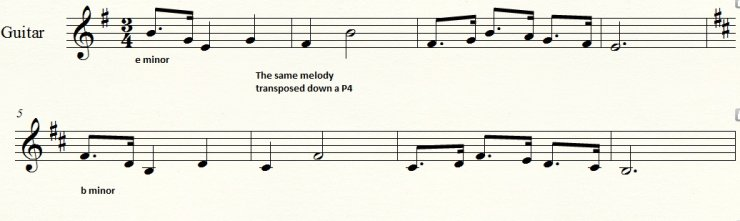## Homework

Homework 3.1
Name the interval given.